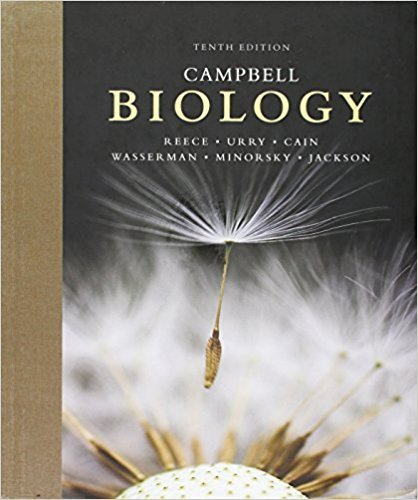×
×

# Based on current knowledge, how would you explain the difference in the percentage ofISBN: 9780321775658 362

## Solution for problem 1 Chapter 20.3

Campbell Biology | 10th Edition

• Textbook Solutions
• 2901 Step-by-step solutions solved by professors and subject experts
• Get 24/7 help from StudySoup virtual teaching assistantsCampbell Biology | 10th Edition

4 5 1 240 Reviews
29
3
Problem 1

Based on current knowledge, how would you explain the difference in the percentage of tadpoles that developed from the two kinds of donor nuclei in Figure 20.16?

Step-by-Step Solution:
Step 1 of 3

Chapter 4: The Light 1. Light is fast more than we know a. Nature of light: Mechanical Light Transport Energy A little energy and it in packet of goes the electric energy *it same way as...

Step 2 of 3

Step 3 of 3

##### ISBN: 9780321775658

This textbook survival guide was created for the textbook: Campbell Biology, edition: 10. This full solution covers the following key subjects: . This expansive textbook survival guide covers 313 chapters, and 1466 solutions. Since the solution to 1 from 20.3 chapter was answered, more than 213 students have viewed the full step-by-step answer. The answer to “Based on current knowledge, how would you explain the difference in the percentage of tadpoles that developed from the two kinds of donor nuclei in Figure 20.16?” is broken down into a number of easy to follow steps, and 27 words. The full step-by-step solution to problem: 1 from chapter: 20.3 was answered by , our top Science solution expert on 03/09/18, 06:51PM. Campbell Biology was written by and is associated to the ISBN: 9780321775658.

Unlock Textbook Solution